#### You may also like### Sometimes We Lose Things

Well now, what would happen if we lost all the nines in our number system? Have a go at writing the numbers out in this way and have a look at the multiplications table.### Nim-like Games

A collection of games on the NIM theme### A Story about Absolutely Nothing

This article for the young and old talks about the origins of our number system and the important role zero has to play in it.

# It Must Be 2000

##### Age 7 to 11 Challenge Level:

We're going to have a look at this number - $2000$.

We've used all sorts of numbers in the past, so let's use our detective skills to find things out about this number $2000$.

It looks like $2000$ because we count in tens and along in a straight line:-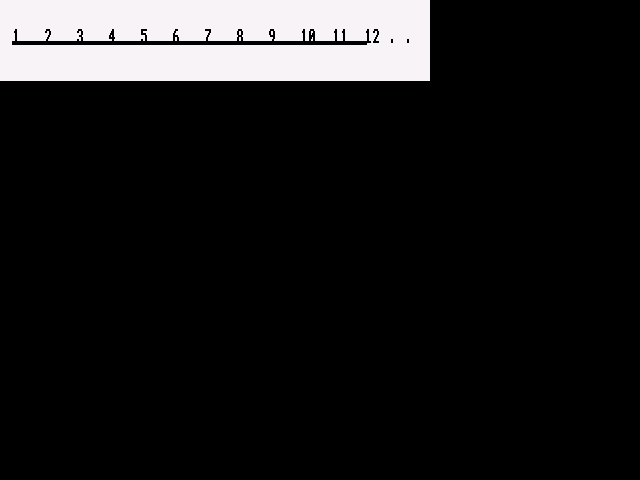Sometimes, yes some "TIMES" we count in a circle.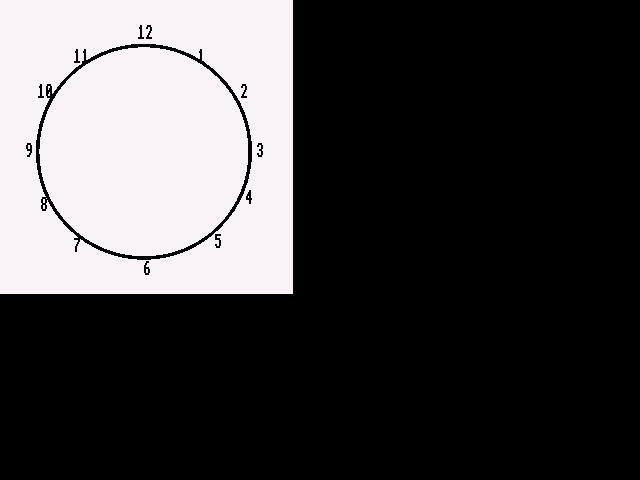If we started counting around this "$12$" clock and counted up to $2000$ we would end up at the number $8$.

But of course we could count in a circle of any size with a different number of numbers around it.

So if the "clock" looked like this:and we counted up to $18$ before returning to $1$, then we would end up at $2$ if we counted to $2000$.

Thinking about it is all to do with remainders. Like this:

$2000$ divided by $12$ will be $166$ remainder $8$

$2000$ divided by $18$ will be $111$ remainder $2$

So we could just look at remainders.

Well save your brains!! I used a spreadsheet on my computer and got the following table.

Column A is the number of numbers around the clock, or the modulo number.

Column B is the remainder when divided into $2000$!

And just for fun I added column A to column B to get column C.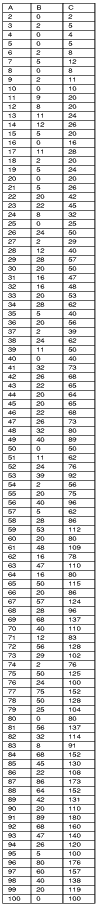Just explore and explore ... Be a detective ... Look, think ... write what you notice and what you think.

Some of you may have also thought about working in different bases. We usually work in base ten, and you may have explored base $2$.
If you are counting $2000$ dots then the number looks like this :-

 Base Number $2$ $110110000$ $3$ $2202002$ $4$ $133100$ $5$ $31000$ $6$ $13133$ $7$ $5555$ $8$ $3720$ $9$ $2662$ and $10$ $20004 Well, what an interesting collection of$3$s and$1$s in bases$4$,$5$and$6$. I found base$7$to be a very big surprise. I have not looked deeply into this, I thought I'd leave it up to you. We're always asking "I wonder what would happen if I ...?" So what if the triangles were made differently? Like this one:-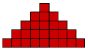Then, for my final contribution, and eagerly waiting to receive your contributions, I offer you this splendid picture:-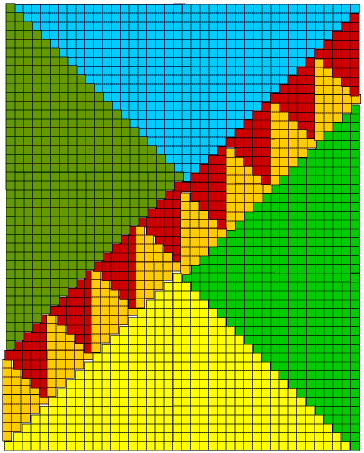This has four large triangles, the$20$th in their sequence each with an area of$400$. There are$16$small triangles, the$5$th in their sequence each with an area of$25$. So you see the total area is$2000\$!